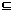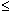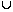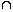## PROBABILITY

1. Experiment:
An operation which can produce some well-defined outcomes is called an experiment.
2. Random Experiment:
An experiment in which all possible outcomes are know and the exact output cannot be predicted in advance, is called a random experiment.
Examples:
1. Rolling an unbiased dice.
2. Tossing a fair coin.
3. Drawing a card from a pack of well-shuffled cards.
4. Picking up a ball of certain colour from a bag containing balls of different colours.
Details:
1. When we throw a coin, then either a Head (H) or a Tail (T) appears.
2. A dice is a solid cube, having 6 faces, marked 1, 2, 3, 4, 5, 6 respectively. When we throw a die, the outcome is the number that appears on its upper face.
3. A pack of cards has 52 cards.
It has 13 cards of each suit, name Spades, Clubs, Hearts and Diamonds.
Cards of spades and clubs are black cards.
Cards of hearts and diamonds are red cards.
There are 4 honours of each unit.
There are Kings, Queens and Jacks. These are all called face cards.
3. Sample Space:
When we perform an experiment, then the set S of all possible outcomes is called thesample space.
Examples:
1. In tossing a coin, S = {H, T}
2. If two coins are tossed, the S = {HH, HT, TH, TT}.
3. In rolling a dice, we have, S = {1, 2, 3, 4, 5, 6}.
4. Event:
Any subset of a sample space is called an event.
5. Probability of Occurrence of an Event:
Let S be the sample and let E be an event.
Then, ES.P(E) = n(E) . n(S)
6. Results on Probability:
1. P(S) = 1
2.P (E)1
3. P() = 0
4. For any events A and B we have : P(AB) = P(A) + P(B) - P(AB)
5. If A denotes (not-A), then P(A) = 1 - P(A).
Example:

What is the probability of getting a sum 9 from two throws of a dice?

In two throws of a die, n(S) = (6 x 6) = 36.
Let E = event of getting a sum ={(3, 6), (4, 5), (5, 4), (6, 3)}.P(E) = n(E) = 4 = 1 . n(S) 36 9
Example:

Three unbiased coins are tossed. What is the probability of getting at most two heads?

Here S = {TTT, TTH, THT, HTT, THH, HTH, HHT, HHH}
Let E = event of getting at most two heads.
Then E = {TTT, TTH, THT, HTT, THH, HTH, HHT}.P(E) = n(E) = 7 . n(S) 8

Example:

Tickets numbered 1 to 20 are mixed up and then a ticket is drawn at random. What is the probability that the ticket drawn has a number which is a multiple of 3 or 5?

Here, S = {1, 2, 3, 4, ...., 19, 20}.
Let E = event of getting a multiple of 3 or 5 = {3, 6 , 9, 12, 15, 18, 5, 10, 20}.P(E) = n(E) = 9 . n(S) 20

Example:
A bag contains 2 red, 3 green and 2 blue balls. Two balls are drawn at random. What is the probability that none of the balls drawn is blue?

Total number of balls = (2 + 3 + 2) = 7.
Let S be the sample space.
Then, n(S)= Number of ways of drawing 2 balls out of 7
7C2 `
 = (7 x 6) (2 x 1)
= 21.
Let E = Event of drawing 2 balls, none of which is blue.n(E)= Number of ways of drawing 2 balls out of (2 + 3) balls.
5C2
 = (5 x 4) (2 x 1)
= 10.P(E) = n(E) = 10 . n(S) 21

Example:

Two dice are tossed. The probability that the total score is a prime number is:

Clearly, n(S) = (6 x 6) = 36.
Let E = Event that the sum is a prime number.
 Then E = { (1, 1), (1, 2), (1, 4), (1, 6), (2, 1), (2, 3), (2, 5), (3, 2), (3, 4), (4, 1), (4, 3),       (5, 2), (5, 6), (6, 1), (6, 5) }n(E) = 15.P(E) = n(E) = 15 = 5 . n(S) 36 12

#### Unknown

Do You Like This??? Then Hit Subscribe Button. You Will Get Every Post, Which Is Worth Reading